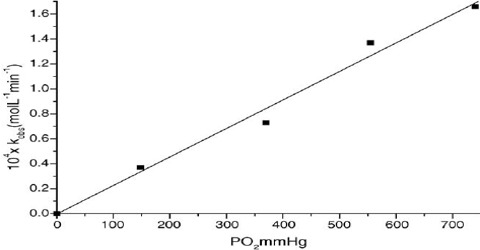Chemistry

# Dependence of Rate on Concentration: The Rate LawDependence of Rate on Concentration: The Rate Law

Experiments show that the rate of a reaction depends on the concentration of one or more of the reactants (and also of catalysts). For a reaction in which the rate is dependent on the concentration of the reactant ‘A’ the experimental results can be expressed as;

rate ∞ cn

This can be expressed as: rate = k cn … … … (1)

Where ‘k’ is a constant of proportionality and is called the rate constant (or the specific reaction rate when unit concentration is considered), ‘c’ is the concentration of ‘A’ and ‘n’ is a constant for that reaction and is called the order of the reaction. The rate constant varies with temperature but is independent of concentration. Equation (1) is known as the rate law or the rate equation for the reaction. The rate constant ‘k’ is defined as the proportionality constant between the rate and the concentration term (concentration with its power). One can also see that rate constant k is equal to the rate when the concentration is mol L-1. The order of the reaction tells us the exact relation between the rate and the concentration. The order of a reaction can be defined as the power to which the concentration is to be raised in order to make the concentration term (concentration with its power) proportional to the rate.

In general for a reaction, A + B → product/products, in which the rate is dependent on the concentrations of the reactants A and B we can write:-

rate = k CmA CnB

where,

• m- is called the order with respect to A
• n- is called the order with respect to B
• m + n is called the overall order of the reaction
• CA is the concentration of A
• CB is the concentration of B